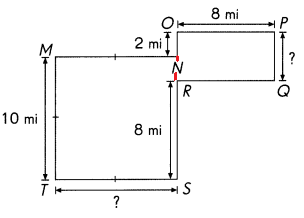# Math in Focus Grade 4 Cumulative Review Chapters 9 to 11 Answer Key

This handy Math in Focus Grade 4 Workbook Answer Key Cumulative Review Chapters 9 to 11 detailed solutions for the textbook questions.

## Math in Focus Grade 4 Cumulative Review Chapters 9 to 11 Answer Key

Concepts and Skills

Name the given angles in another way. (Lesson 9.1)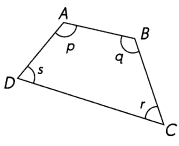Question 1.
∠p : _____________
The another way of angle ∠p : ∠DAB or ∠BAD

Question 2.
∠r : _____________
The another way of angle ∠r : ∠BCD or ∠DCB

Question 3.
∠ABC : _____________
The another way of angle ∠ABC : ∠q or ∠CBA

Question 4.
The another way of angle ∠ADC : ∠S or ∠CDA

Estimate and decide which of the above angle measures are (Lesson 9.1)

Question 5.
acute angles.
The estimated acute angles are  ∠BCD and ∠CDA

Question 6.
obtuse angles.
The estimated obtuse angles are ∠DAB and ∠BAD.

Estimate each angle measure. Then measure each angle to check your answer. (Lesson 9.1)

Question 7.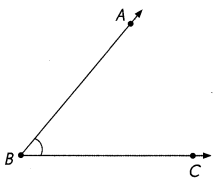Measure of ∠ABC = _____________
The estimated measure of  ∠ABC =45°
After measuring, the actual measure of angle  ∠ABC =50°

Question 8.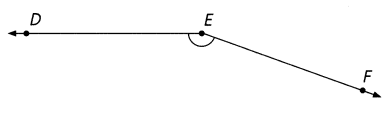Measure of ∠DEF = ______________
The estimated measure of  ∠DEF =135°
After measuring, the actual measure of angle ∠DEF =150°

Estimate each angle measure. Then measure each angle to check your answer. (Lesson 9.1)

Question 9.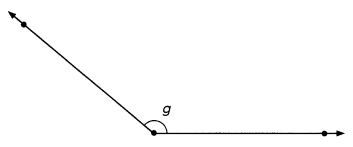Measure of ∠g ____________
The estimated measure of  ∠g =135°
After measuring, the actual measure of angle ∠g =135°

Question 10.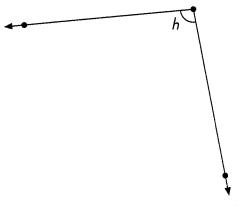Measure of ∠h _____________
The estimated measure of  ∠h =110°
After measuring, the actual measure of angle ∠h =105°

Name and measure each marked angle in the figure. (Lesson 9.2)

Question 11.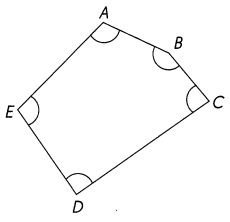Example
Measure of ∠BAE = 110°
Measure of _____________
Measure of _____________
Measure of _____________
Measure of _____________
Measure of ∠BAE = 110°
Measure of ∠AED = 100°
Measure of ∠EDC = 90°
Measure of ∠DCB = 85°
Measure of ∠CBA = 135°

Using point A as the vertex draw ∠CAB as described. (Lesson 9.2)

Question 12.
75°, with $$\overrightarrow{A C}$$ above $$\overrightarrow{A B}$$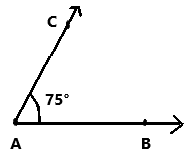Question 13.
42°, with $$\overrightarrow{A C}$$ below $$\overrightarrow{A B}$$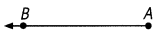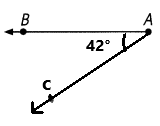Question 14.
105°, with $$\overrightarrow{A C}$$ above $$\overrightarrow{A B}$$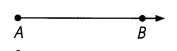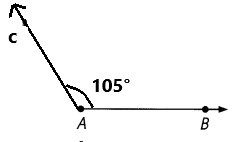Question 15.
127°, with $$\overrightarrow{A C}$$ below $$\overrightarrow{A B}$$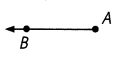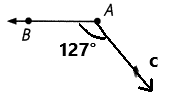Fill in the blanks. (Lesson 9.3)

Question 16.
$$\frac{3}{4}$$ of a full turn is __________.
3/4 of a full turn is 270°.

Question 17.
Two right angles is ________ of a full turn.
Two right angles is 1/2 of a full turn.

Question 18.
360° is ____________ full turn or ___________ right angles.
360° is one full turn or four right angles.

Question 19.
What fraction of a full turn is one right angle? ____________
1/4 fraction of a full turn is one right angle.

Draw. $$\overleftrightarrow{A B}$$ is a vertical line. (Lessons 10.1 to 10.3)

Question 20.
Draw a horizontal line through point B and label it $$\overleftrightarrow{B C}$$.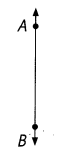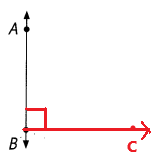Drawn a horizontal line through point B and labeled as BC.

Question 21.
Draw a vertical line through point C and label it $$\overleftrightarrow{C D}$$.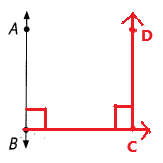Drawn a vertical line through point C and labeled as CD.

Question 22.
What can you say about the relationship between $$\overleftrightarrow{A B}$$ and $$\overleftrightarrow{B C}$$?
The relationship between both AB and BC are perpendicular to each other.

Question 23.
What can you say about the relationship between $$\overleftrightarrow{A B}$$ and $$\overleftrightarrow{C D}$$?
The relationship between both AB and CD are parallel to each other.

Use a drawing triangle and a straightedge. (Lessons 10.1 and 10.2)

Question 24.
Draw a line segment parallel to $$\overline{P Q}$$ through point R.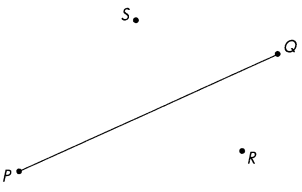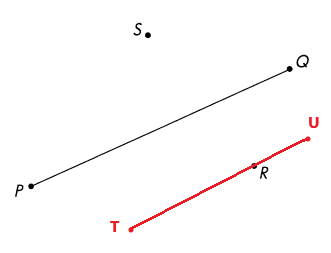Drawn a line segment parallel to PQ through point R.

Question 25.
Draw a line segment perpendicular to $$\overline{P Q}$$ through point S.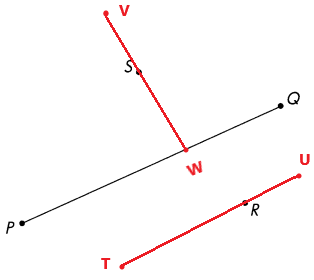Drawn a line segment perpendicular to PQ through point S.

Fill in the blanks. (Lesson 11.1)

Question 26.
ABCD is a square.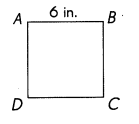BC = __________ in.
CD = __________ in.
ABCD is a square.
BC = 6 in.
CD = 6 in.
A square is a plane figure with four equal straight sides and four right angles.

Question 27.
PQRS is a rectangle.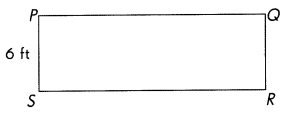$$\overline{S R}$$ is 3 times as long as $$\overline{P S}$$.
SR = _________ ft
PQ = _________ ft
SR is 3 times as long as PS.
From the above figure we can  observe PS= 6ft
SR = 3 × PS
SR = 3 x 6 feet
SR = 18 feet
PQ = 18 feet
A Rectangle is a four sided-polygon, having all the internal angles equal to 90 degrees. The two sides at each corner or vertex, meet at right angles. The opposite sides of the rectangle are equal in length which makes it different from a square.

Find the measures of the unknown angles in the squares and rectangles. (Lesson 11.2)

Question 28.
STUV is a square.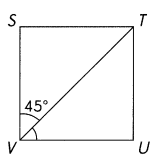Measure of ∠TVU = _____________
Measure of ∠TVU = 45°.

Question 29.
ABCD is a rectangle.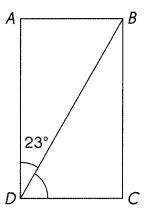Measure of ∠BDC = _____________
Measure of ∠BDC = 67°

Question 30.
MNOP is a rectangle.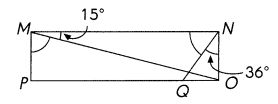Measure of ∠MNQ = ___________
Measure of ∠OMP = _____________
Measure of ∠MNQ = 54°
Measure of ∠OMP = 75°

Question 31.
PQRS is a square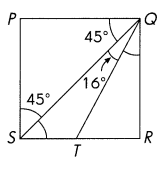Measure of ∠QSR = ____________
Measure of ∠RQT = _____________
Measure of ∠QSR = 45°
Measure of ∠RQT = 29°

Solve. All sides in the figures meet at right angles. Find the lengths of the unknown sides in each figure. (Lesson 11.2)

Question 32.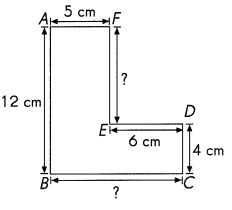EF = ____________ cm
BC = ____________ cm
EF = AB – CD
EF = 12 cm – 4 cm
EF = 8 cm
BC = DE + FA
BC = 6 cm + 5 cm
BC = 11 cm

Question 33.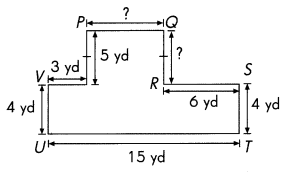QR = ____________ yd
PQ = ____________ yd
From the above figure we know that WP = 5 yd
QR = WP
QR = 5 yd
From the above figure we know that VW = 3 yd, RS = 6 yd, UT = 15 yd
PQ = UT – VW – RS
PQ = 15 – 3 – 6
PQ = 6 yd

Question 34.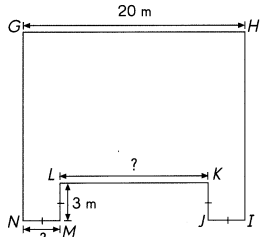NM = ____________ m
LK = _____________ m
From the above figure we know that ML = 3m
NM = ML
NM = 3 m
From the above figure we know that HG = 20 m, NM = 3m, JI = 3m
LK = HG – NM – JI
LK = 20 – 3 – 3
LK = 14 m

Question 35.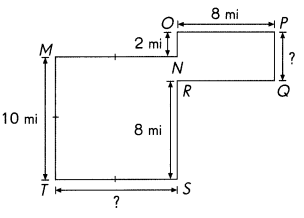PQ = _____________ mi
TS = _____________ mi